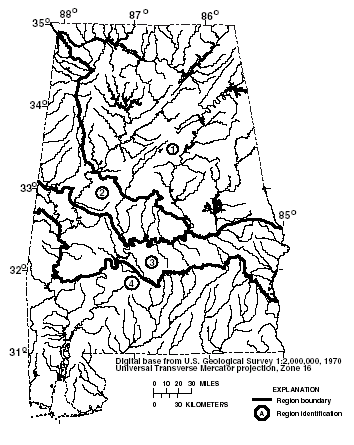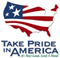# The National Flood-Frequency Program—Methods for Estimating Flood Magnitude and Frequency in Rural and Urban Areas in Alabama

#### U.S. Geological Survey Fact Sheet 088-97 June, 1999

This report is available in pdf 635KB.

# Introduction

Estimates of the magnitude and frequency of flood-peak discharges and flood hydrographs are used for a variety of purposes, such as for the design of bridges, culverts, and flood-control structures; and for the management and regulation of flood plains. To provide simple methods of estimating flood-peak discharges, the U.S. Geological Survey (USGS) has developed and published equations for every State, the Commonwealth of Puerto Rico, and a number of metropolitan areas in the United States. In 1993, the USGS, in cooperation with the Federal Emergency Management Agency and the Federal Highway Administration, compiled all current USGS statewide and metropolitan area equations into a computer program, titled “The National Flood-Frequency (NFF) Program” (Jennings and others, 1994).

Since 1993, new or updated equations have been developed by the USGS for various areas of the Nation. These new equations have been incorporated into an updated version of the NFF Program.

Fact sheets that describe application of the updated NFF Program to various areas of the Nation are available. This fact sheet describes the application of the updated NFF Program to streams that drain rural and urban areas in Alabama.

## Rural Basins

Alabama is divided into four hydrologic regions (fig. 1) on the basis of geology, physiography, and analyses of flood-frequency distributions. Atkins (1996) developed regression equations for estimating peak discharges (QT), in cubic feet per second, that have recurrence intervals (T) that range from 2 to 500 years for ungaged, unregulated, rural streams. The regression equations are not applicable to streams in urban areas, which were defined by Atkins (1996) as sites in which more than 5 percent of the drainage area is covered by impervious surfaces, or regulated or channelized streams.Figure 1. Hydrologic regions for Alabama

Contributing drainage area (A) is the most statistically significant explanatory watershed variable and the only explanatory watershed variable that is used in the regression equations. Contributing drainage area is the total area that contributes runoff upstream of the stream site of interest. Contributing drainage areas were measured in square miles for the equations determined by Atkins (1996), but the NFF Program will accept input and report results in either the inch-pound or metric system of units.

The regression equations, the average standard errors of prediction, the equivalent years of record, and the range of the drainage area that is applicable for use in the equations are shown in table 1. The average standard error of prediction is a measure of the average accuracy of a regression equations when used to estimate peak-discharge values for ungaged watersheds similar to those that were used to derive the regression equations. The equivalent years of record is the number of years of streamflow record needed to achieve the same accuracy as the regression equation. The standard error of prediction increases appreciably when drainage-area values that are outside the quoted range of data used to develop the equations are applied in the equation.

Regression equation Average standard error Equivalent Applicable range of of prediction, years of record drainage area, in percent in square miles Table 1. Flood-peak discharge regression equations and associated statistics for streams that drain rural areas in Alabama (modified from Atkins, 1996) [QT, peak discharge for recurrence interval T, 2 to 500 years, in cubic feet per second; A, contributing drainage area; Do., ditto] Q2 =227A0.672 35 3 0.44–1,027 Q5 = 374A0.669 34 4 Do. Q10 = 482A0.669 35 5 Do. Q25 = 627A0.668 37 7 Do. Q50 = 739A0.667 39 7 Do. Q100 = 855A0.667 41 8 Do. Q200 = 974A0.666 43 9 Do. Q500 = 1,135A0.666 46 9 Do. Q2 = 163A0.664 40 3 0.13–831 Q5 = 295A0.654 36 4 Do. Q10 = 406A0.648 35 6 Do. Q25 = 573A0.640 35 9 Do. Q50 = 716A0.634 36 10 Do. Q100 = 877A0.628 37 11 Do. Q200 = 1,057A0.622 39 12 Do. Q500 = 1,327A0.614 43 12 Do. Q2 = 347A0.573 37 3 0.44–1,097 Q5 = 517A0.607 35 5 Do. Q10 = 638A0.626 34 7 Do. Q25 = 796A0.648 33 10 Do. Q50 = 914A0.663 33 12 Do. Q100 = 1,032A0.677 33 15 Do. Q200 = 1,148A0.689 34 17 Do. Q500 = 1,302A0.704 35 18 Do. Q2 = 169A0.616 38 3 1.44–1,344 Q5 = 313A0.608 33 6 Do. Q10 = 444A0.600 33 9 Do. Q25 = 650A0.592 35 12 Do. Q50 = 831A0.587 38 13 Do. Q100 = 1,035A0.583 42 13 Do. Q200 = 1,262A0.579 46 13 Do. Q500 = 1,601A0.576 52 13 Do.

When the drainage area of the site of interest is in more than one region, a weighted estimate of the peak discharge should be computed. The equations for the appropriate regions should be applied independently using basinwide estimates of the required explanatory variables as if the entire basin was in each region. The weighted estimate is then computed by multiplying each regional estimate against the fraction of the drainage area in that region and summing the products. The NFF Program provides an algorithm for this computation.

The regression equations were developed by using peak-discharge data for streamflow-gaging stations with at least 10 years of record collected through September 1991 at 198 locations in Alabama and 72 locations in Florida, Georgia, Mississippi, and Tennessee. Atkins (1996) summarized observed station-record, peak-discharge estimates, and regression-weighted estimates for 270 streamflow-gaging stations in Alabama and parts of adjacent States. Atkins (1996) also presented graphs of peak discharge as a function of drainage area for selected recurrence intervals for the Alabama, the Black Warrior, the Cahaba, the Choctawhatchee, the Conecuh, and the Tombigbee Rivers.

## Urban Basins

Olin and Bingham (1982) developed regression equations for estimating urban peak discharges (QT) that have recurrence intervals (T) that range from 2 to 100 years (table 2). The explanatory basin variables used in the equations are contributing drainage area (A), in square miles, and the percentage of the basin that is covered by impervious area (IA). These variables can be estimated from topographic maps; IA can also be estimated from aerial photographs. The applicable range of basin variables used to develop the equations and the standard errors of estimate are summarized in table 2. The standard error of estimate is the standard deviation of the regression residuals and is a measure of the accuracy of a regression equation applied to the data used to develop the regression.

Regression equation Averagestandarderror of estimate, Applicable Applicable range of range of percent, in percent drainage area, impervious area in square miles Table 2. Flood-peak discharge regression equations and associated statistics for streams that drain urban areas in Alabama (from Olin and Bingham, 1982) [QT, peak discharge for recurrence interval T, 2 to 100 years, in cubic feet per second; A, contributing drainage area; IA, impervious area; do., Do., ditto] Q2 = 150A0.70IA0.36 26 0.16-83.5 8.3-42.9 Q5 = 210A0.70 IA0.39 24 do. Do. Q10 = 266A0.69 IA0.39 24 do. Do. Q25 = 337A0.69 IA0.39 24 do. Do. Q50 = 396A0.69 IA0.38 25 do. Do. Q100 = 444A0.69 IA0.39 25 do. Do.

The urban equations are not applicable to region 3 (fig. 1), which is underlain by impervious chalk and marl. Caution should be used in estimating flood magnitudes in basins underlain by soluble limestone and in basins with sinkholes, quarries, or storage reservoirs. Such features can be identified by study of geologic and topographic maps or by reconnaissance of the basin. The equations do not apply to urban streams that are subject to temporary in-channel storage or overbank storage. For basins that contain less than 5 percent impervious area, the rural equations should be used.

## References

Atkins, J.B., 1996, Magnitude and frequency of floods in Alabama: U.S. Geological Survey Water-Resources Investigations Report 95–4199, 234 p.

Jennings, M.E., Thomas, W.O., Jr., and Riggs, H.C., comps., 1994, Nationwide summary of U.S. Geological Survey regional regression equations for estimating magnitude and frequency of floods for ungaged sites, 1993: U.S. Geological Survey Water-Resources Investigations Report
94–4002, 196 p.

Olin, D.A., and Bingham, R.H., 1982, Synthesized flood-frequency of urban streams in Alabama: U.S. Geological Survey Water-Resources Investigations Report 82–683, 23 p.

U.S. Geological Survey

Office of Surface Water

415 National Center

Reston, Virginia 20192

(703) 648-5301

USGS hydrologic analysis software is available for electronic retrieval through the World Wide Web (WWW) at: http://water.usgs.gov/software/ and through anonymous File Transfer Protocol (FTP) from water.usgs.gov (directory:/pub/software). The WWW page and FTP directory from which the National Flood-Frequency software and user documentation can be retrieved are http://water.usgs.gov/software/nff.html and /pub/software/surface_water/nff, respectively.

Additional earth science information is available from the USGS through the WWW at http://www.usgs.gov/ or by calling 1-888-ASK-USGS.

 AccessibilityFOIAPrivacyPolicies and Notices U.S. Department of the Interior, U.S. Geological Survey Persistent URL: Page Contact Information: Contact USGS Last modified: Tuesday, November 29 2016, 05:53:36 PM